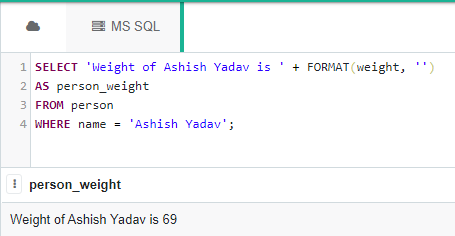# SQL Query to Convert FLOAT to NVARCHAR

Here we will see, how to convert FLOAT data to NVARCHAR data in an MS SQL Server’s database table using the CAST(), CONVERT(), and FORMAT() functions.

We will be creating a person table in a database called “geeks”.

### Creating the Database:

`CREATE DATABASE geeks;`

### Using the Database:

`USE geeks;`

### Table Definition:

We have the following Employee table in our geeks database :

```CREATE TABLE person(
id INT IDENTITY(1,1) PRIMARY KEY,
name VARCHAR(30) NOT NULL,
weight REAL NOT NULL);```

You can use the below statement to query the description of the created table:

`EXEC SP_COLUMNS person;`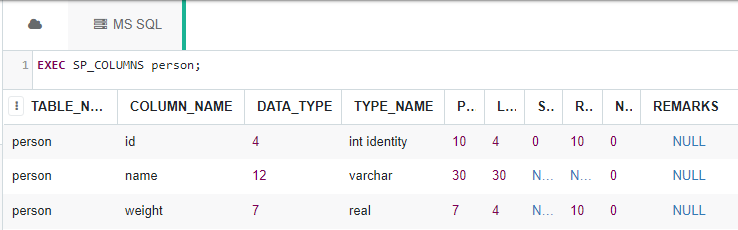Use the below statement to add data to the person table:

```INSERT INTO person
VALUES
('Yogesh Vaishnav', 62.5),
('Vishal Vishwakarma', 70),

To verify the contents of the table use the below statement:

`SELECT * FROM person;`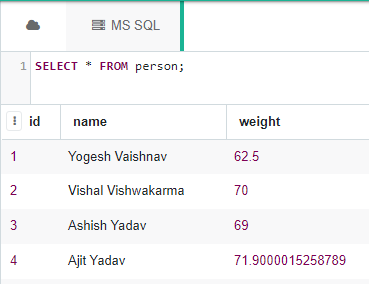Now let’s convert FLOAT values to nvarchar using three different methods.

### Using the CONVERT() function:

```Syntax: SELECT CONVERT(<DATA_TYPE>, <VALUE>);
--DATA_TYPE is the type we want to convert to.
--VALUE is the value we want to convert into DATA_TYPE.```

Example:

```SELECT 'Weight of Yogesh Vaishnav is ' + CONVERT(NVARCHAR(20), weight)
AS person_weight
FROM person
WHERE name = 'Yogesh Vaishnav';```

Output: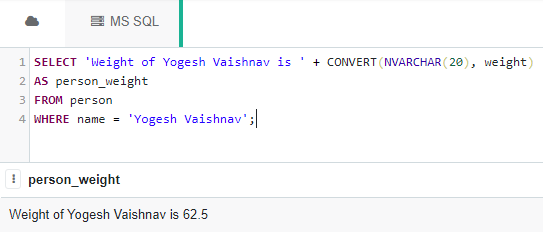### Using the CAST() function:

```Syntax: SELECT CAST(<VALUE> AS <DATA_TYPE>);
--DATA_TYPE is the type we want to convert to.
--VALUE is the value we want to convert into DATA_TYPE.```

Example:

```SELECT 'Weight of Yogesh Vaishnav is ' + CAST(weight as NVARCHAR(20))
AS person_weight
FROM person
WHERE name = 'Yogesh Vaishnav';```

Output: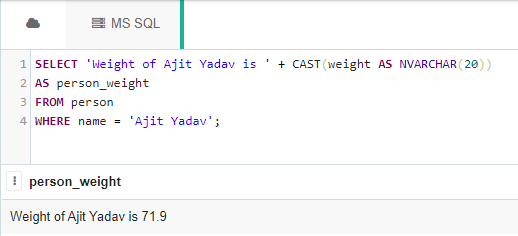### Using the FORMAT() function:

Although the FORMAT() function is useful for formatting datetime and not converting one type into another, still can be used to convert(or here format) float value into an STR value.

```Syntax: SELECT FORMAT(<VALUE> , 'actual_format';
--actual_format is the format we want to achieve in a string form.
--VALUE is the value we want to format according to the actual_format.```

Example:

```SELECT 'Weight of Ashish Yadav is ' + FORMAT(weight, '') --'' denotes no formatting
--i.e simply convert it to a string of characters.
AS person_weight
FROM person